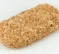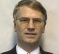## Off by one windowingStarted by 3 years ago13 replieslatest reply 3 years ago165 views

In https://www.dsprelated.com/freebooks/sasp/Bartlett..., there are two variations of a Bartlett/triangular window sized 3:

0 1 0

0.5 1 0.5

However, I think the triangular window of 3 size should look like this:

0 2÷3 2÷3

The windows mentioned in the page look like truncated or expanded versions of power of two triangular window filters:

0 1

0 0.5 1 0.5

I think what happened there is that it was defining the triangular window in terms of a convolution of two rectangular windows of 1.5 size, but in the process of aliasing rounded the rectangular window to either 1 or 2 samples. Therefore, computing a triangular window with convolution is only suitable for even sizes, a Parzen window with convolution only for sizes that are false modulo 4, etc. . No wonder the Parzen window was chosen to be a power of two in amount of convolutions, as it works well for typical power of two fast fourier transforms.

[ - ]Triangle windows are always symmetric around their midpoint (that is the reason they are used).

So for n=3 0 1 0 and for n=4 0 2/3 2/3 0 (or 1/2 1 1/2 and 1/4 3/4 3/4 1/4 respectively).

Y(J)S

[ - ]The index n÷2 will be the midpoint for length n (for being the start point of the second half). Your "n=3 0 1 0" has the correct mid point for length 2, but the start and end point are the same point, so it should be "n=2 0 1". And similarly it should be "n=3 0 2÷3 2÷3". On the other hand, the "0.5 1 0.5" you mentioned lacks the startpoint entirely, so they should be incremented in length, "0 0.5 1 0.5". Whereas in case of "0.25 0.75 0.75 0.25", this would imply the start point at location -0.5 (or 3.5), so it is phase shifted, and in the neutral 'cosine' phase it would be again "0 0.5 1 0.5".

We have the symmetry that for each point i, it is equal to the point n-i (or (-i)%n). For example, for the four point window:

w=0 (equal to w)

w=0.5 (equal to w)

w=1 (equal to w)

w=0.5 (equal to w)

Looks symmetrical to me.

[ - ]OK, now I understand what you are saying. You assumed that there is overlap between the last point and the first point, so that there are not 2 zeroed-out points in a row when one uses non-overlapping blocks.

That is not the assumption usually made. The window is assumed to work on 1:N or 0:(N-1) NOT on 0:N with periodicity of N. Even when we assume periodic (AKA cylindrical) boundary conditions when we say N points we mean N points not N-1 points.

We often use overlap of 25% or 50% and where the 2 zeroes-in-a-row issue is a non-issue. But even when there is no overlap the whole idea is to zero out the endpoints to avoid boundary discontinuities.

All that said, for any reasonable N the difference will be utterly negligible. Don't try to improve your spectral properties by fooling around with the triangular window. Use a better window.

[ - ]I haven't said I am using triangular window for practical uses. Also there is no better or worse window, we have various use cases that might necessitate use of different windowing filters.

With a window of n samples I do mean n samples: Hann of 6 is '0 0.25 0.75 1 0.75 0.25'.

A periodic window makes a lot of sense because a fast fourier transform is periodic on a fundamental level. Also, because periodic windowing has no off-by-one error, it will actually make the 25% overlap or 50% overlap work properly. This is actually an interesting property of cosine sum filters. When we have Hann/Hamming with terms of 0 and 1, it is suitable for overlap of 1÷2, but also 1÷3, 1÷4, etc., if we have terms of 0, 1, and 2, like in Blackman window, then 1÷2 won't work but 1÷3 and 1÷4 will, and in general case, we can have 1÷n overlap as long as there is no cosine sum term m where m is true and m%n is false, so for 1÷4 overlap we can have terms 0, 1, 2, 3, 5, 6, 7, 9, etc. .

[ - ]What you say makes sense, but as I said in my previous reply, for any reasonable N the difference in straightforward usage is completely negligible (for any window type).

An issue worth checking is the building of complex structures from smaller basic ones of restricted sizes, like when using the Winograd transform or number transforms. Perhaps you could build some structure where this really makes a difference.

[ - ]I'm not sure if you're aware that we commonly use at least 2 different symmetries in window functions. You mentioned (or implied) that you don't have a practical application in mind, but the application will ultimately determine which symmetry is suitable.

In filter design, we use windows that are symmetric about their midpoint. In spectral analysis (e.g. calculating power spectra), we use a sort of "periodic" sampling to match the implied periodicity in a DFT.

The periodic version is equivalent to calculating an (N+1)-point symmetric window and discarding the last value. This makes sense if you imagine computing the DFT of a pure sinewave. We don't want the last value of the sine "window" to be 0, because then when the waveform is repeated periodically, we'll get two consecutive zeros (which is wrong).

Many (but not all) MATLAB windowing functions offer the choice between these two variants by passing a 'symmetric' or 'periodic' flag into the function. For example, see sflag here:
https://uk.mathworks.com/help/signal/ref/blackmanh...

[ - ]I would rather think of it the opposite way. A symmetric window is equivalent to an off-by-one error in a periodic window.

[ - ]"symmetric window from periodic window or periodic from symmetric" sounds like chicken/egg puzzle.

But I am more unimpressed by the "rectangular window" which is not a window. Moreover it has other names. Apologies but aren't we wasting too much time on such puzzles.

[ - ]A rectangular window is indeed a windowing filter, because it still filters a particular section of time,

[ - ]I would say if a new concept (term) helps then that is good idea...otherwise it is adding noise and misleading.

A first paper I read on digital filters listed the very first case as y(n) = x(n) and regarded it as a filter that passes its input unchanged with unity gain. then moved on to y(n) = -x(n), then y(n) = 2*x(n), before moving on to proper filter (first order IIR).

These are not filters and are not a subset of filters but are headache agents. It is wasting space and reader's time. It is like saying any wiring is a filter...great

[ - ]A rectangular windowing isn't infinite response though. A rectangular windowing filter only passes through a specific range of time by truncating it, filtering out all content outside.

[ - ]Is there a typo here

"0 2÷3 2÷3" ?

"0 1÷3 2÷3", i.e. ~ "0 0.333333 0.666666" ?

If not, maybe a slight expansion of the text to tease out more of the 'obvious' aspects you discuss, so that it's easier to see where the issue(s) lie.

[ - ]A triangular window slopes up in the first half, then down in the second half. So, the 20 point window would be:

0 0.1 0.2 0.3 0.4 0.5 0.6 0.7 0.8 0.9 1 0.9 0.8 0.7 0.6 0.5 0.4 0.3 0.2 0.1

The 6 point window:

0 1÷3 2÷3 1 2÷3 1÷3

The 3 point window:

0 2÷3 2÷3

You could think of it as an n-point window being: for (i=0; i<n; i++) the point i is 1-abs((i÷n)×2-1). So, a 3-point window has the following i÷n: 0÷3, 1÷3, 2÷3, and their resulting window points are 0, 2÷3, 2÷3.